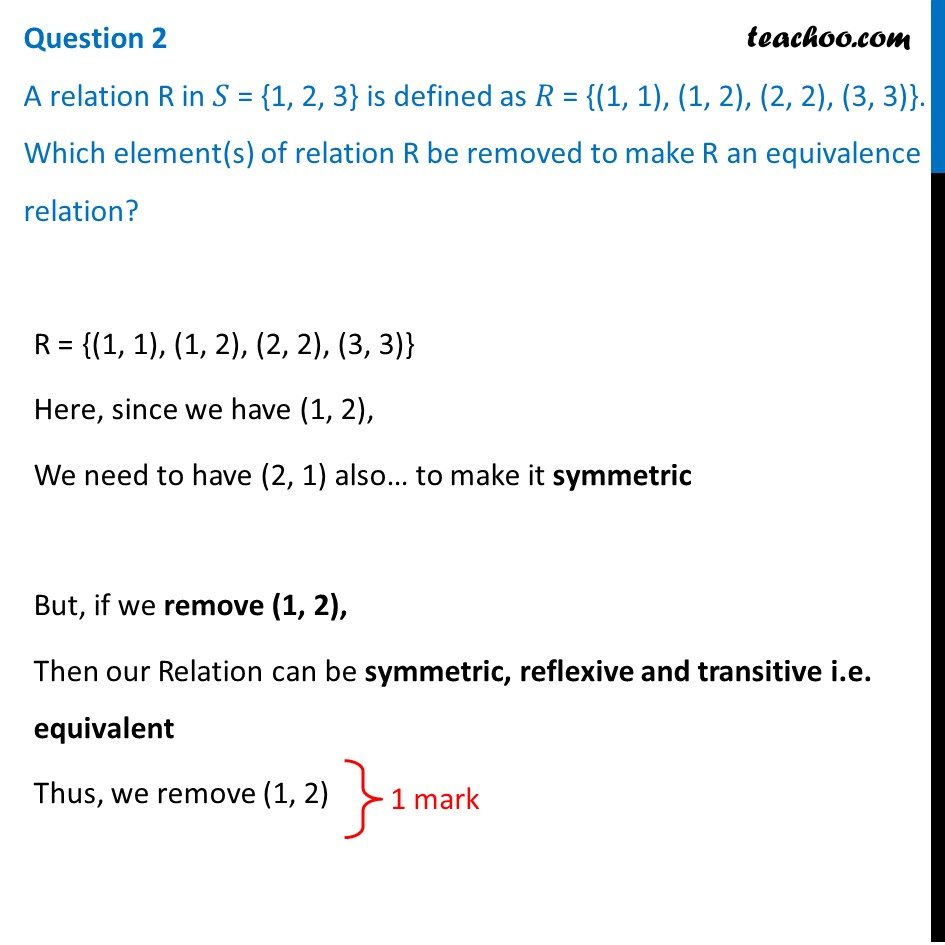## A relation R in 𝑆 = {1,2,3} is defined as 𝑅 = {(1, 1), (1, 2), (2, 2), (3, 3)}. Which element(s) of relation R be removed to make R an equivalence relation?1. Class 12
2. Solutions of Sample Papers and Past Year Papers - for Class 12 Boards
3. CBSE Class 12 Sample Paper for 2021 Boards

Transcript

Question 2 A relation R in 𝑆 = {1, 2, 3} is defined as 𝑅 = {(1, 1), (1, 2), (2, 2), (3, 3)}. Which element(s) of relation R be removed to make R an equivalence relation? R = {(1, 1), (1, 2), (2, 2), (3, 3)} Here, since we have (1, 2), We need to have (2, 1) also… to make it symmetric But, if we remove (1, 2), Then our Relation can be symmetric, reflexive and transitive i.e. equivalent Thus, we remove (1, 2)

CBSE Class 12 Sample Paper for 2021 Boards

Class 12
Solutions of Sample Papers and Past Year Papers - for Class 12 Boards